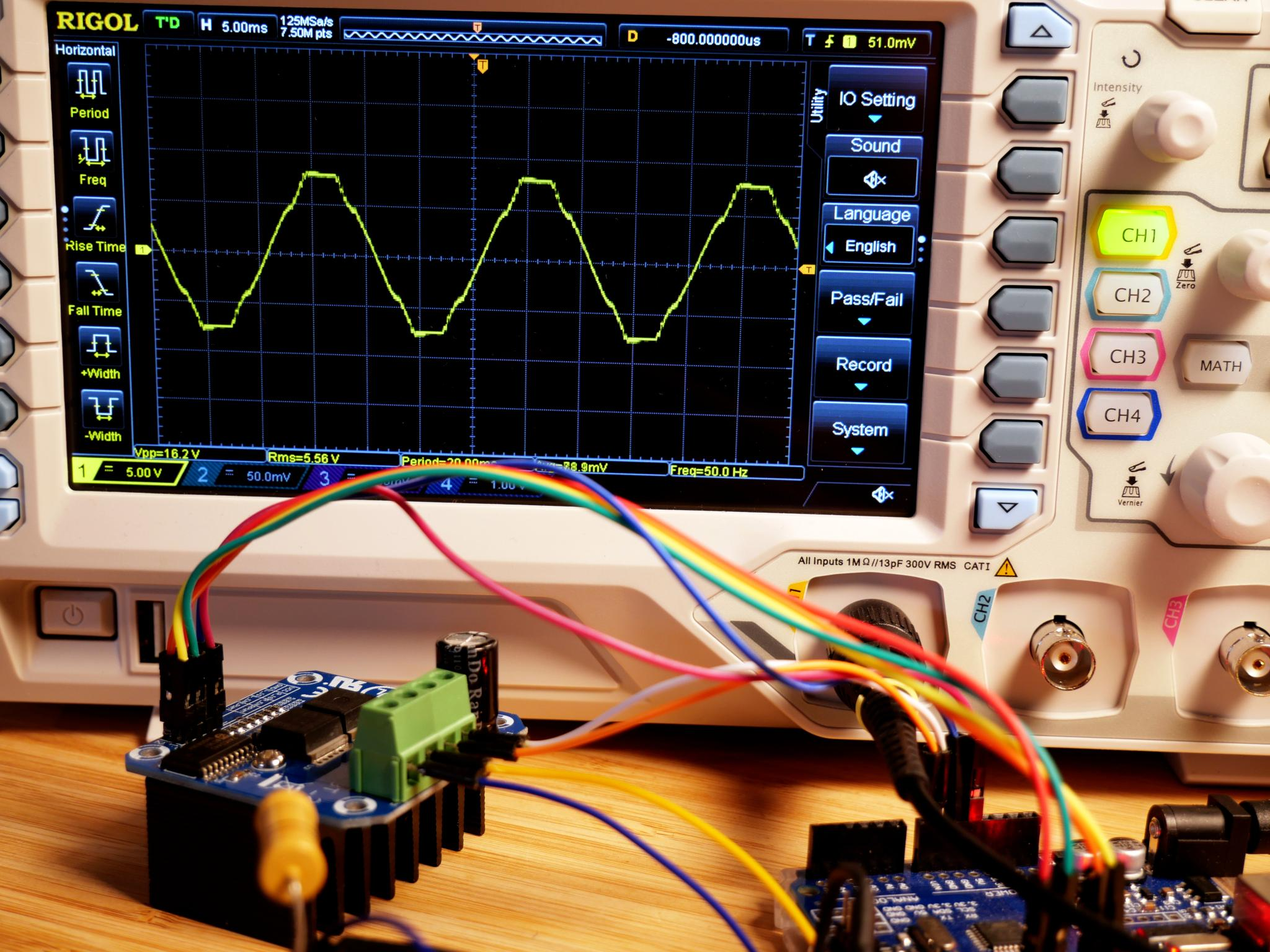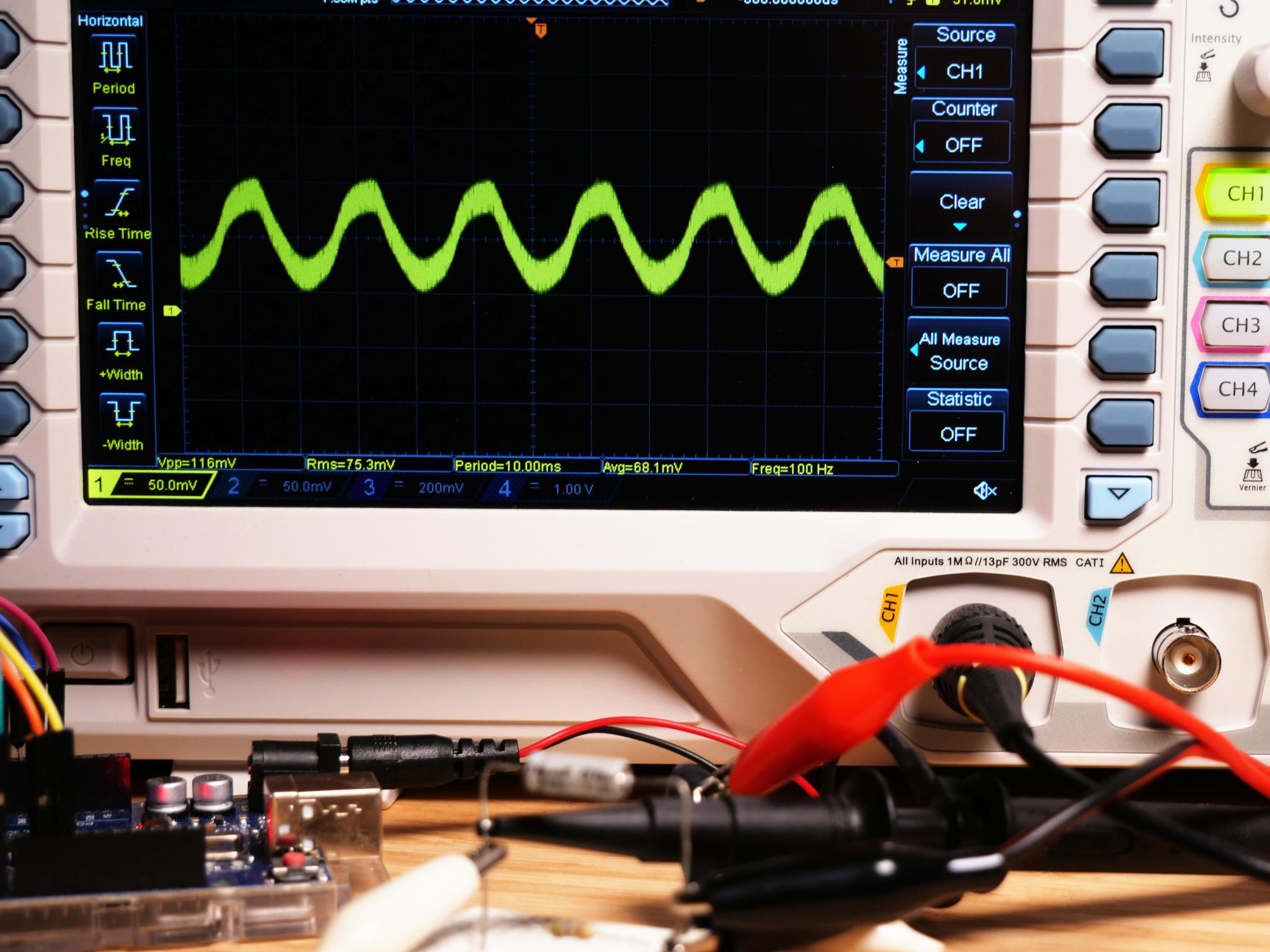# Low Voltage AC Source (Part 10)

Last time we built an AC source using a transistor H-bridge. This time, we replace the transistors with MOSFETs to improve the efficiency.

## Increasing the Efficiency with a MOSFET H-Bridge

Last time we build an H-bridge inverter circuit using transistors. This solution turned out simpler to build than the previous solutions which required complex amplification circuits. H-bridge inverters can be very efficient, however, ours was definitely not. One major issue was the high amount of base current through the transistors and their high power dissipation. Both issues can be solved by replacing the transistors with MOSFETs, which is exactly what we are going to do today.

MOSFETs and other field effect transistors (FETs) are voltage-controlled, whereas the classic bipolar junction transistors (BJTs) are current-controlled. Since FETs work differently than BJTs, they also use a different naming scheme. However, in principle, the gate (G) roughly corresponds to the transistors base (B). The collector (C) corresponds to the FETs drain (D), and the emitter (E) to the source (S). Similar to normal transistors there are n- and p-channel MOSFETs. However, when using MOSFETs there is no current flow through the gate, like there is through the base of a transistor. Additionally, MOSFETs typically have a much lower internal resistance and thus power dissipation than transistors. This is especially beneficial if MOSFETs are used as switches. Both properties will help us to boost the efficiency of our circuit.

With all these advantages, why not always use MOSFETs? Well, MOSFETs are not only more difficult to build and thus more expensive, they also have some downsides. The first one being, that they do conduct in reverse polarity situations. An n-channel MOSFETs acts like a diode if you attach a positive voltage to its source pin. In schematics this is often visualized by drawing an additional diode across the MOSFET. Depending on the application this behavior is a problem or not. For our circuit this isn't an issue. More important for us is the fact that a lot of MOSFETs require a relatively high threshold voltage to fully turn on. Not all MOSFETs can be directly used with the 5 V of the Arduino Uno. However, the most significant issue for us is that the gate of MOSFETs, especially if they are big, has a rather large capacitance. This means that one has to either accept slow switching speeds, which we can't since we use PWM, or charge and discharge the gate with a high current. For the latter, you normally need a MOSFET driver. In our case, however, we are going to use a ready-made MOSFET H-bridge module using the BTN7960.

## Building the Circuit

Let's start by building up the circuit. Due to using the ready-made module it is less complex than the previous ones. It consists only of the Arduino, the MOSFET H-bridge and an LC-filter with a 51 Ω load.

The MOSFET module is intended as a motor driver and uses two BTN7960 MOSFET half-bridges. The module implements everything you need for driving motors. It additionally comes with an over-temperature, over-voltage, under-voltage and short-circuit protection. It also has an internal dead-time generator, so that we don't need to implement that in the Arduino program anymore.

However, the module also has its limitations. Since it is intended as a motor driver, it does not require very fast switching speeds. The limit is at 25 kHz. This means that we need to reduce the frequency of our PWM signal. Additionally, the module requires more than the Arduino's 5 V power supply. The ideal supply voltage is between 8 V and 18 V. The module starts to work at 5.5 V, however, 5 V are insufficient. At 5 V the under-voltage protection triggers during load peaks and disables the MOSFETs. It is not possible to generate a stable sine wave. To use the module, we need an external power supply. You can use a lab bench supply or a 9V battery for this purpose. Luckily, the module comes with an integrated logic-level converter, so that we can use the Arduino's 5 V for the control signals.

To connect the module to the Arduino Uno connect the PWM pins 9 and 10 to RPWM and LPWM. Additionally, we need to enable the MOSFETs. For this we are going to use the pins 6 and 7 and connect them to R_EN and L_EN. For powering the module the Arduino's 5V need to be connected to the VCC pin of the logic-level converter. The VIN pin with the higher voltage needs to be connected to the screw terminal B+. It is used to power the MOSFETs. The output signal, which needs to be connected to the LC filter and the load, is available at M+ and M-.# The Code

Let's have a look at the code. It is pretty much the same as for the transistor H-bridge except that we don't need the dead-time generation any longer. There are two new parts that require some explanation. But first, here is the full code on one view:

#include <EEPROM.h>

// PWM frequency is 7.8 kHz so we can update roughly every 256 us in phase correct mode
const int usPerCommand = 300;

// Precalculated Voltage Buffer
const int BUFFER_SIZE = 256;
unsigned int voltages[BUFFER_SIZE];
unsigned int steps;
unsigned int usPerStep;

unsigned int current_step = 0;
unsigned long start_time;

// Setup frequency
void setup() {
Serial.begin(9600);
Serial.print("Enter Frequency (Hz): ");

// Wait 10s for input otherwise take stored value
Serial.setTimeout(10000);
float frequency = Serial.parseFloat();
if(frequency == 0) EEPROM.get(0, frequency);
else EEPROM.put(0, frequency);

Serial.println(frequency);

// Calculate number of possible steps
int possible_steps = 1000000/usPerCommand/frequency;

// Steps need to be a multiple of 4 to keep the sine form
steps = (possible_steps  / 4) * 4;
if(steps > BUFFER_SIZE) steps = BUFFER_SIZE;
if(steps < 4) steps = 4;

// Time per Step
usPerStep = 1000000 / (frequency * steps);
if(usPerStep < usPerCommand) usPerStep = usPerCommand;

// Precalculate Sine Values 0-5 V
for(int i = 0; i < steps; i++) {
int value = sin(i*3.14*2/steps) * 255 + 255;
voltages[i] = constrain(value, 0, 1022);
}

Serial.print("Number of output steps: ");
Serial.println(steps);

Serial.print("Microseconds per step: ");
Serial.println(usPerStep);

Serial.print("Archieved Frequency (Hz): ");
Serial.println(1000000.0/float(steps)/float(usPerStep));

// Enable a prescaler of 1 for timer 1 but count to 1023
// choose phase correct mode and invert output 10
TCCR1A = (1<<WGM11)|(1 << COM1A1)|(1 << COM1B1)|(1 << COM1B0);
TCCR1B = (1<<CS10);
TIMSK1 |= (1<<TOIE1);

// Enable PWM pins
pinMode(9, OUTPUT);
pinMode(10, OUTPUT);

// Enable MOSFETs
pinMode(6, OUTPUT);
pinMode(7, OUTPUT);
digitalWrite(6, HIGH);
digitalWrite(7, HIGH);

// Initially set start time
start_time = micros();
}

ISR(TIMER1_OVF_vect) {
OCR1A = voltages[current_step];
OCR1B = voltages[current_step];
}

// Output values
void loop() {
current_step++;
if(current_step >= steps) current_step = 0;

while(micros()-start_time < usPerStep);
start_time += usPerStep;
}

What is new? Well, the first obvious change is that we now configure pins 6 and 7 as outputs and set them to HIGH which activates the MOSFETs. Nothing special or worth explaining about that. The more difficult part is the one for the PWM signal generation. Here we have some changes to reduce the frequency of the PWM signal to match the limits of the MOSFET module.

Instead of the original 31.25 kHz we now use a frequency of 7.8 kHz. This is a reduction by a factor of four and can be achieved by letting the counter that produces the PWM signal count from 0 to 1023 instead of 0 to 255. For this I reconfigured the timer using the following lines of code:


// Enable a prescaler of 1 for timer 1 but count to 1023
// choose phase correct mode and invert output 10
TCCR1A = (1<<WGM11)|(1 << COM1A1)|(1 << COM1B1)|(1 << COM1B0);
TCCR1B = (1<<CS10);
TIMSK1 |= (1<<TOIE1);

The disadvantage of this solution is, that we can't use the predefined analogWrite function any longer. For this reason we now need to configure the PWM pins 9 and 10 as output manually and set the target value manually within the timer interrupt, as it is shown below.

ISR(TIMER1_OVF_vect) {
OCR1A = voltages[current_step];
OCR1B = voltages[current_step];
}

# The Result

How does our result look like? The sine wave gets slightly distorted by the MOSFET module. This can be fixed by reducing the amplitude, as the distortion only happens near to the maximum and minimum value. However, in this part of the tutorial we care more about the output power and efficiency than about producing a perfect sine wave, so I don't want to talk about this any further. The output power at an effective output voltage of roughly 5.5 V is:

$$P_{out} = {U^2 \over R} = {{5.5 V}^2 \over 51 Ω} \approx 0.6 W$$

Is this the maximum output power? No, certainly not. In my case, the limiting factor is the high resistance of the coil. At higher currents it would overheat and fail. With a proper coil for higher currents a much higher output power can be achieved.What about the efficiency? To measure the efficiency I measured the overall current consumption. For this I used a small 0.56 Ω resistor and a 1 uF capacitor for smoothing in parallel. I put both in series to the Arduino and measured the voltage drop with the oscilloscope. As you can see in the image below it was 68 mV in average which equals to an input current of roughly 120 mA.

$$I = {U \over R} = {68 mV \over 0.56 Ω} \approx 120 mA$$This corresponds to an input power of 1.08 W for powering both the Arduino and our actual circuit.

$$P_{in} = U \cdot I = 9 V \cdot 120 mA = 1.08 W$$

The overall efficiency is thus roughly 56 %, which is more than we achieved with any of our previous solutions. A respectable result, considering, that we are misusing a motor driver to build an inverter circuit and loosing a lot of energy due to the high resistance of the coil. We also have to keep in mind that we included the power consumption of the Arduino in this calculation. In the efficiency calculations I did for the circuits with amplifiers I didn't to that, because I didn't measure but instead calculated a theoretical efficiency value for them.

$$\eta = {P_{out} \over P_{in}} = {0.6 W \over 1.08 W} \approx 56 \%$$